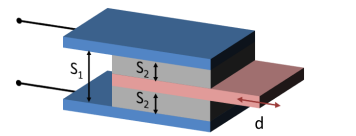### Create an Account

Already have account?

### Forgot Your Password ?

Home / Questions / Capacitors can be used in sensing applications such as fuel gauges in airplanes and vibrat...

# Capacitors can be used in sensing applications such as fuel gauges in airplanes and vibration sensors The following is an application where a capacitive sensor can be used to find displacement d The

Capacitors can be used in sensing applications such as fuel gauges in airplanes and vibration sensors. The following is an application where a capacitive sensor can be used to find displacement d. The area of the plates is 4 cm x 4 cm. S1 = 2.5mm, S2 = 1mm. The area between the plates is filled with air which has a relative permittivity of 1 a. Find the expression the relates total capacitance with the displacement d

b. Find the displacement if the total capacitance is 6.181 x 10^-12 F## 1 Approved Answer

Jul 27 2020 View more View Less

#### Answer (Solved)Subscribe To Get Solution